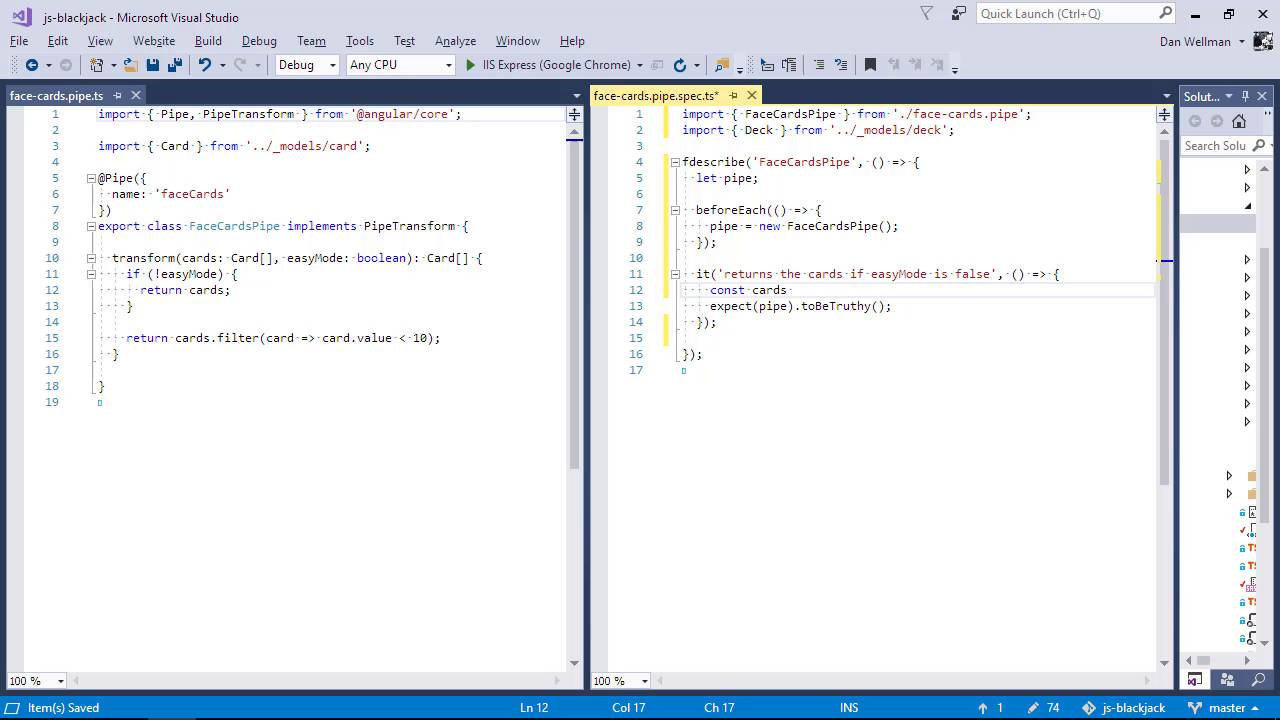Lessons: 67Length: 8.9 hours• Overview
• Transcript

# 8.6 Unit Testing Pipes

In this lesson you'll see how we can unit test the transform method of a custom pipe that we've created ourselves.

## 10.Conclusion1 lesson, 01:32

### 8.6 Unit Testing Pipes

Hi, folks. In this lesson, we're going to see how we can unit test pipes in Angular. We did create a pipe earlier in the course, which is used to filter out the face cards in the deck when easy mode is enabled. We create the pipe using the CLI, so we already have the spec file ready to go. Let's open it up as well as the actual file being tested, side by side in the editor and like we have done with our other test files. Great, so first of all, let's focus this test. The pipe only has a single dependency, and the default tests should run and pass as it is. So let's just check that's the case. So we can see that one test ran, and that test passed so we don't need to do any special set up in order to get this test file ready for tests. So let's go ahead and update the existing test. So the existing test was creating an instance of the pipe in order to test it. We're gonna want to do that for every single test. So let's a before each that initializes the pipe before each test. So we just new up an instance of the pipe just like as if we were testing a service. So now let's add the test description. What does the pipe actually do? The pipe returns the cards if easy mode is falsey. It doesn't do anything at all. It just returns the cards that were passed to the transform method. So this aspect of the pipe should be quite straightforward to test. We can just pass false to the pipe, and the returned array of cards should still be 52 in length, so we won't need to bring in the deck for this test So we first get the cards array from a fresh instance of the deck. We then pass this array of cards to the transfer method of the pipe setting easy mode, which is the second parameter true false. And we then check the length of the resulting array that gets passed back from the transform method and we specify that it should equal 52. So let's check the output in the browser. And we can see that that test is passing. So now we can test that when we pass true as the second argument, the pipe does filter out the face cards So the text is going to be very similar to before, we've updated the description. We're still going to want the array of cards, so that doesn't need to change. But now, when we use the transform message, we want to pass through as the second argument instead of false. And this time there should only be 36 cards left in the pack once all of the face cards have been taken out. And let's see if this test is passing. And we can see that it is. So that's really all that we need to test for this particular pipe. This particular pipe is very very simple, but pipes are normally much more complex than this, they can be. And in that case, the spec file would be similarly more complex. But for us, that's what we need to do so in this lesson, we saw how we can test a pipe in an Angular application. Pipes are generally one of the easier parts of an application to test, as they're generally quite small and self-contained. So we just need to create an instance of them and invoke the transform method, and check how that behaves. Thanks for watching.

Back to the top